# Texas Go Math Grade 8 Module 3 Answer Key Proportional Relationships

Refer to our Texas Go Math Grade 8 Answer Key Pdf to score good marks in the exams. Test yourself by practicing the problems from Texas Go Math Grade 8 Module 3 Answer Key Proportional Relationships.

## Texas Go Math Grade 8 Module 3 Answer Key Proportional RelationshipsEssential Question
How can you use proportional relationships to solve real-world problems?

Complete these exercises to review skills you will need for this chapter.

Write each fraction as decimal.

Question 1.
$$\frac{3}{8}$$
To express $$\frac{3}{8}$$ as a decimal, we write the fraction as a division problem.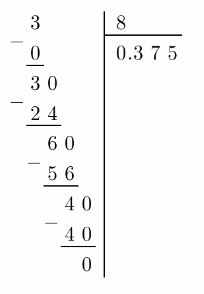Therefore, $$\frac{3}{8}$$ = 0.375

Question 2.
$$\frac{0.3}{0.4}$$
First, multiply the numerator and the denominator by 10 so that the denominator is a whole number.
$$\frac{0.3 \times 10}{0.4 \times 10}$$ = $$\frac{3}{4}$$
Now write the fraction as a division problem, place a decimal point in the quotient and divide as whole numbers:0.75

Question 3.
$$\frac{0.13}{0.2}$$
First multiply the numerator and the denominator by 10 so that the denominator is a whole number.
$$\frac{0.13 \times 10}{0.2 \times 10}$$ = $$\frac{1.3}{2}$$
Now write the fraction as a division problem. place a decimal point in the quotient and divide as whole numbers:0.65

Question 4.
$$\frac{0.39}{0.75}$$
First, multiply the numerator and the denominator by 100 so that the denominator is a whole number.
$$\frac{0.39 \times 100}{0.75 \times 100}$$ = $$\frac{39}{75}$$
Now write the fraction as a division problem, place a decimal point in the quotient and divide as whole numbers: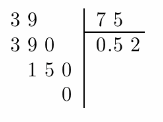0.52

Question 5.
$$\frac{4}{5}$$
Write the fraction as a division problem, place a decimal point in the quotient and divide as whole numbers$$\frac{4}{5}$$ = 0.8

Question 6.
$$\frac{0.1}{2}$$
First, we multiply the numerator and the denominator by a power of 10 so that we get whole numbers.
$$\frac{0.1}{2}$$ = $$\frac{0.1 \cdot 10}{2 \cdot 10}$$ = $$\frac{1}{20}$$
To express $$\frac{1}{20}$$ as a decimal, we write the fraction as a division problem.Therefore,
$$\frac{0.1}{2}$$ = 0.05

Question 7.
$$\frac{3.5}{14}$$
First, we multiply the numerator and the denominator by a power of 10 so that we get whole numbers.
$$\frac{3.5}{14}$$ = $$\frac{3.5 \cdot 10}{14 \cdot 10}$$ = $$\frac{35}{140}$$
To express $$\frac{35}{140}$$ as a decimal, we write the fraction as a division problem.Therefore, $$\frac{3.5}{14}$$ = 0.25

Question 8.
$$\frac{7}{14}$$
To express $$\frac{7}{14}$$ as a decimal, we write the fraction as a division problem.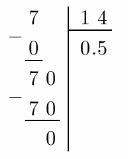$$\frac{7}{14}$$ = 0.5

Question 9.
$$\frac{0.3}{10}$$
First, we multiply the numerator and the denominator by a power of 10 so that we get whole numbers.
$$\frac{0.3}{10}$$ = $$\frac{0.3 \cdot 10}{10 \cdot 10}$$ = $$\frac{3}{100}$$
To express $$\frac{3}{100}$$ as a decimal, we write the fraction as a division problem.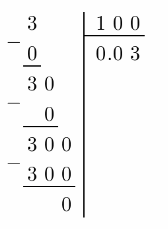$$\frac{0.3}{10}$$ = 0.03

Solve each proportion for x.

Question 10.
$$\frac{20}{18}$$ = $$\frac{10}{x}$$ ______
$$\frac{20}{18}$$ = $$\frac{10}{x}$$ Given
$$\frac{20 \div 2}{18 \div 2}$$ = $$\frac{10}{x}$$ Divide 20 ÷ 2 = 10, so divide the numerator and denominator by 2
$$\frac{10}{9}$$ = $$\frac{10}{x}$$
x = 9 compare

Question 11.
$$\frac{x}{12}$$ = $$\frac{30}{72}$$ ______
$$\frac{x}{12}$$ = $$\frac{30}{72}$$ Given
$$\frac{x}{12}$$ = $$\frac{30 \div 6}{72 \div 6}$$ Divide 72 ÷ 6 = 12, so divide the numerator and denominator by 6.
$$\frac{x}{12}$$ = $$\frac{5}{12}$$
x = 5 compare

Question 12.
$$\frac{x}{4}$$ = $$\frac{4}{16}$$ ______
$$\frac{x}{4}$$ = $$\frac{4}{16}$$ Given
$$\frac{x}{4}$$ = $$\frac{4 \div 4}{16 \div 4}$$ Divide 16 ÷ 4 = 4, so divide the numerator and denominator by 4.
$$\frac{x}{4}$$ = $$\frac{1}{4}$$
x = 1 compare

Question 13.
$$\frac{11}{x}$$ = $$\frac{132}{120}$$ ______
$$\frac{11}{x}$$ = $$\frac{132}{120}$$ Given
$$\frac{11}{x}$$ = $$\frac{132 \div 12}{120 \div 12}$$ Divide 132 ÷ 12 = 11, so divide the numerator and denominator by 12.
$$\frac{11}{x}$$ = $$\frac{11}{10}$$
x = 10 compare

Question 14.
$$\frac{36}{48}$$ = $$\frac{x}{4}$$ ______
$$\frac{36}{48}$$ = $$\frac{x}{4}$$ Given
$$\frac{36 \div 12}{48 \div 12}$$ = $$\frac{x}{4}$$ Divide 48 ÷ 12 = 4, so divide the numerator and denominator by 12.
$$\frac{3}{4}$$ = $$\frac{x}{4}$$
x = 3 compare

Question 15.
$$\frac{x}{9}$$ = $$\frac{21}{27}$$ ______
$$\frac{x}{9}$$ = $$\frac{21}{27}$$ Given
$$\frac{x}{9}$$ = $$\frac{21 \div 3}{27 \div 3}$$ Divide 27 ÷ 3 = 9, so divide the numerator and denominator by 12.
$$\frac{x}{9}$$ = $$\frac{7}{9}$$
x = 7 Compare
x = 7

Question 16.
$$\frac{24}{16}$$ = $$\frac{x}{2}$$ ______
$$\frac{24}{16}$$ = $$\frac{x}{2}$$ Given
$$\frac{24 \div 8}{16 \div 8}$$ = $$\frac{x}{2}$$ Divide 16 ÷ 8 = 2, so divide the numerator and denominator by 8.
$$\frac{3}{2}$$ = $$\frac{x}{2}$$
x = 3 Compare
x = 3

Question 17.
$$\frac{30}{15}$$ = $$\frac{6}{x}$$ ______
$$\frac{30}{15}$$ = $$\frac{6}{x}$$ Given
$$\frac{30 \div 5}{15 \div 5}$$ = $$\frac{6}{x}$$ Divide 30 ÷ 5 = 6, so divide the numerator and denominator by 5.
$$\frac{6}{3}$$ = $$\frac{6}{x}$$
x = 3 Compare
x = 3

Question 18.
$$\frac{3}{x}$$ = $$\frac{18}{36}$$ ______
$$\frac{3}{x}$$ = $$\frac{18}{36}$$
$$\frac{3}{x}$$ = $$\frac{18 \div 6}{36 \div 6}$$ Divide 18 ÷ 6 = 3, so divide the numerator and denominator by 6.
$$\frac{3}{x}$$ = $$\frac{3}{6}$$
x = 6

Visualize Vocabulary
Use the ✓ words to complete the diagram.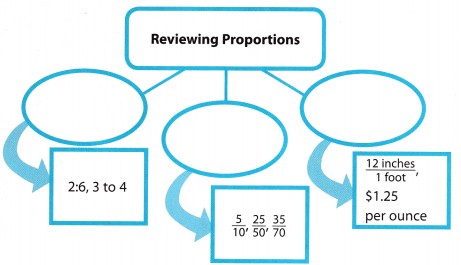Understand Vocabulary

Match the term on the left to the definition on the right.